### 零知识证明 Learn by Coding：libsnark 入门篇

libsnark 是目前实现 zk-SNARKs 电路最重要的框架，在众多私密交易或隐私计算相关项目间广泛应用，其中最著名当然要数 Zcash。Zcash 在 Sapling 版本升级前一直使用 libsnark 来实现电路（之后才替换为 bellman）。毫不夸张地说，libsnark 支撑并促进了 zk-SNARKs 技术的首次大规模应用，填补了零知识证明技术从最新理论到工程实现间的空缺。

## 1. zk-SNARKs 和 libsnark 背景简介

zk-SNARKs 能应用到很多场景，比如隐私保护、区块链扩容、可验证计算等。本文不介绍 zk-SNARKS 和零知识证明的理论细节，不熟悉或想深入了解的同学可阅读其他文章或论文。

• [GGPR13] Quadratic span programs and succinct NIZKs without PCPs , Rosario Gennaro, Craig Gentry, Bryan Parno, Mariana Raykova, EUROCRYPT 2013
• [PGHR13] Pinocchio: Nearly Practical Verifiable Computation , Bryan Parno, Craig Gentry, Jon Howell, Mariana Raykova, IEEE Symposium on Security and Privacy (Oakland) 2013
• [BCGTV13] SNARKs for C: Verifying Program Executions Succinctly and in Zero Knowledge , Eli Ben-Sasson, Alessandro Chiesa, Daniel Genkin, Eran Tromer, Madars Virza, CRYPTO 2013
• [BCIOP13] Succinct non-interactive arguments via linear interactive Proofs , Nir Bitansky, Alessandro Chiesa, Yuval Ishai, Rafail Ostrovsky, Omer Paneth, Theory of Cryptography Conference 2013
• [BCTV14a] Succinct non-interactive zero knowledge for a von Neumann architecture , Eli Ben-Sasson, Alessandro Chiesa, Eran Tromer, Madars Virza, USENIX Security 2014
• [BCTV14b] Scalable succinct non-interactive arguments via cycles of elliptic curves , Eli Ben-Sasson, Alessandro Chiesa, Eran Tromer, Madars Virza, CRYPTO 2014
• [Groth16] On the Size of Pairing-based Non-interactive Arguments , Jens Groth, EUROCRYPT 2016

libsnark 的开发者们亦是这个领域内顶尖的学者或研究牛人，如 Eran Tromer 更是以上多篇论文的共同作者。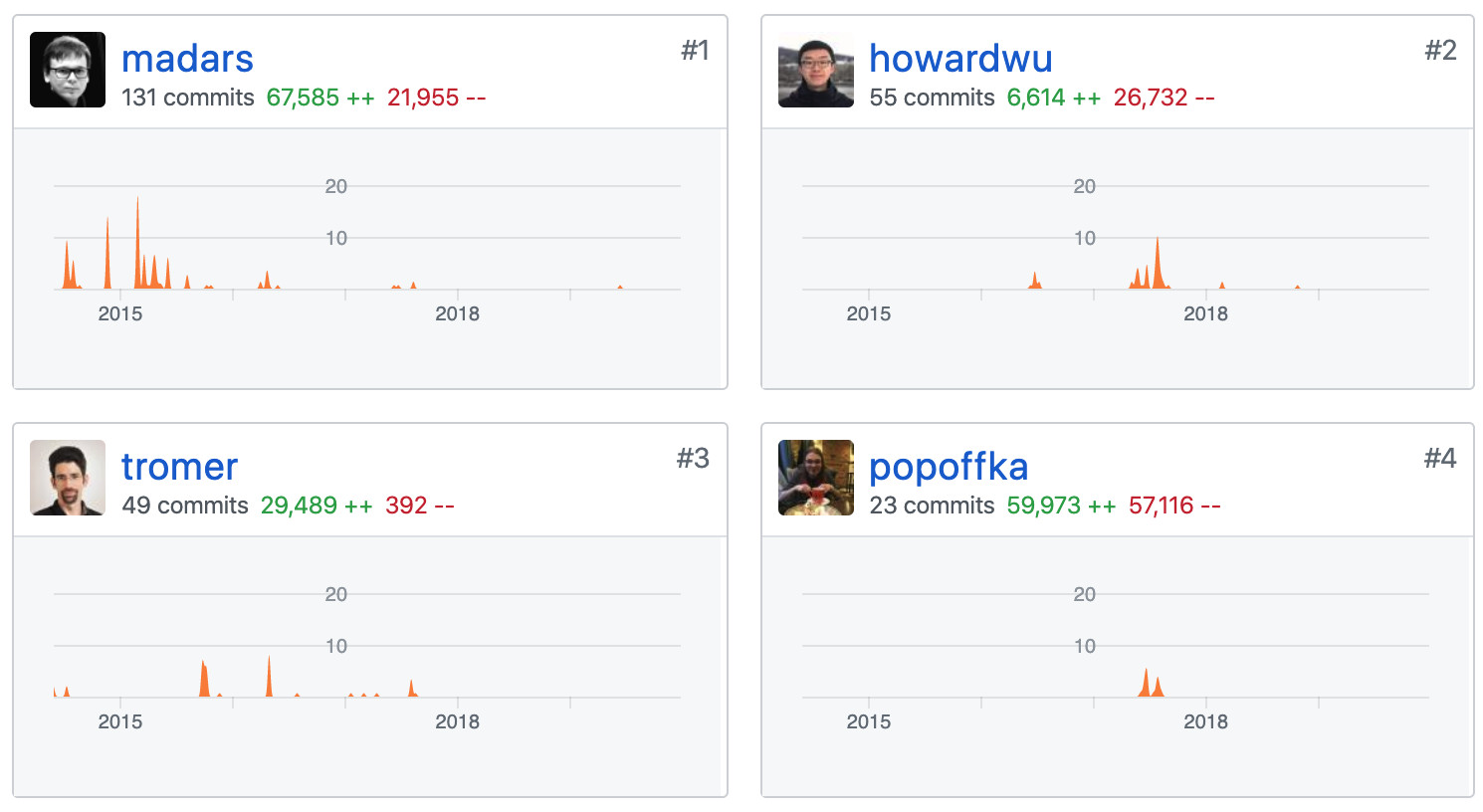扎实的理论基础和工程能力，让 libsnark 的作者们能够化繁为简，将形如下图的高深理论和复杂公式逐一实现，高度工程化地抽象出简洁的接口供广大开发者方便地调用。向这些将非凡的理论研究推广至更大规模应用的先锋们致敬。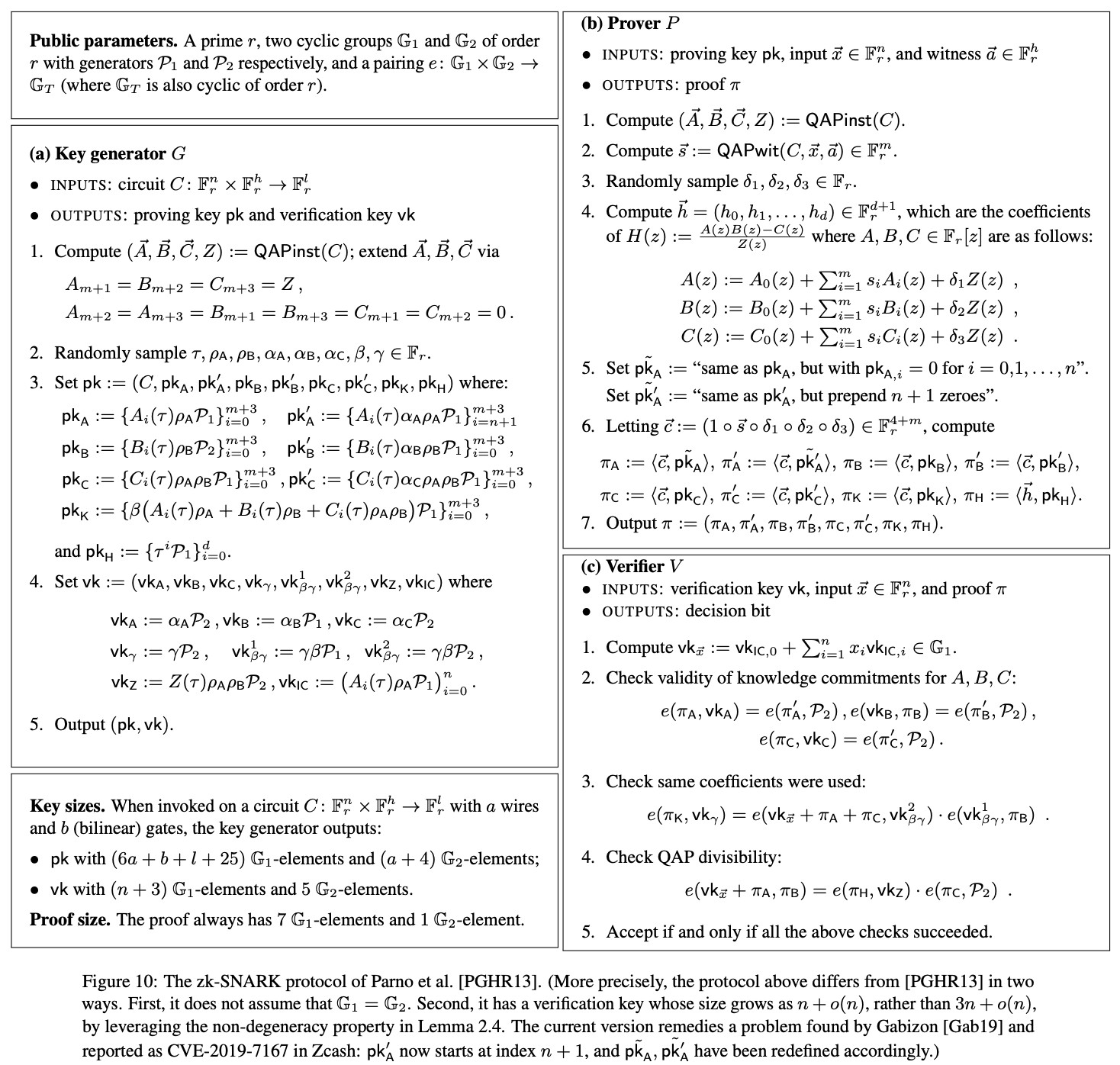下图是 libsnark 的模块总览图，摘自 libsnark 代码贡献量第一作者 Madars Virza 在 MIT 的博士论文。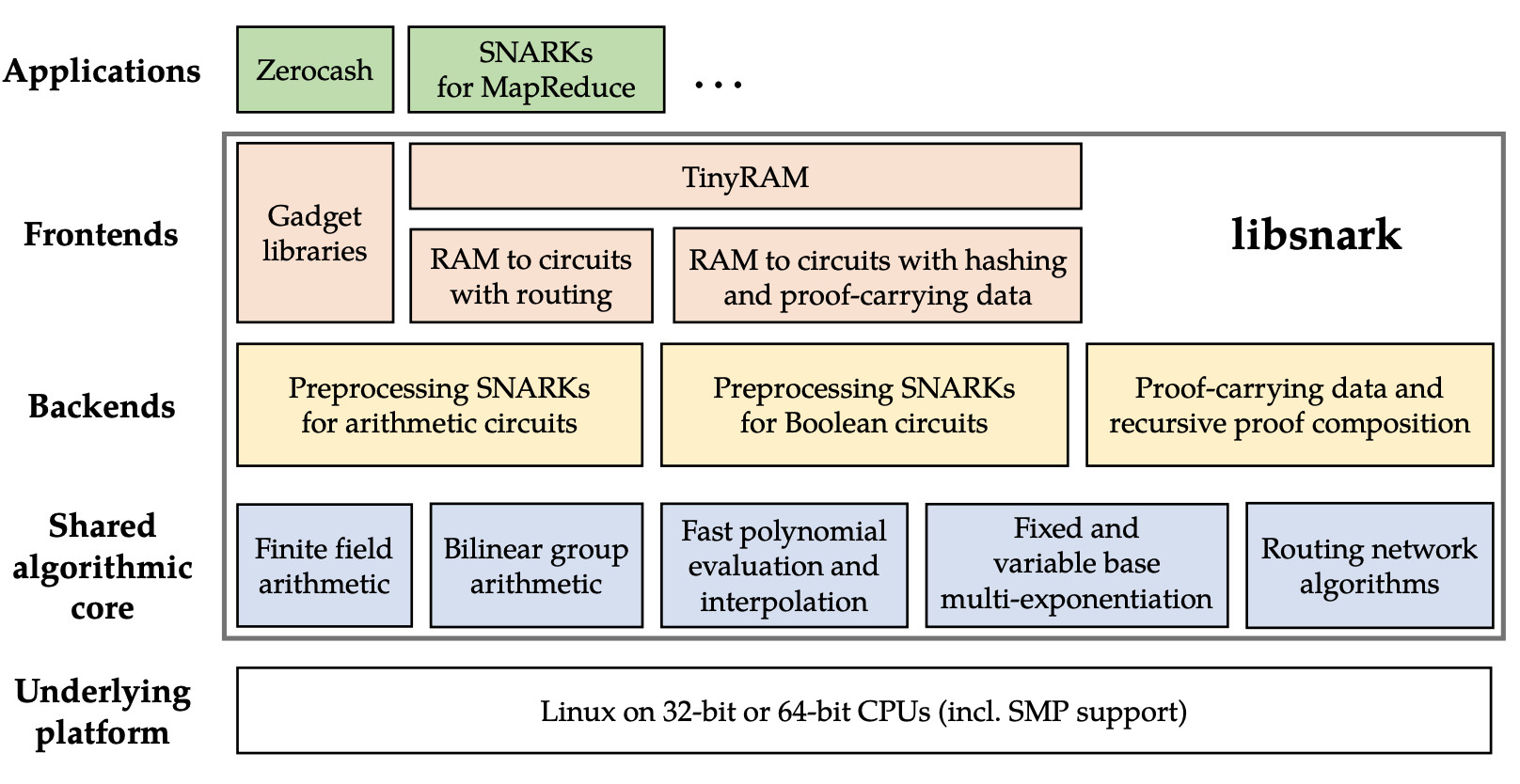libsnark 框架提供了多个通用证明系统的实现，其中使用较多的是 BCTV14a 和 Groth16。

• zk_proof_systems/ppzksnark/r1cs_ppzksnark 对应的是 BCTV14a
• zk_proof_systems/ppzksnark/r1cs_gg_ppzksnark 对应的是 Groth16

## 2. 基本原理与步骤

1. 将待证明的命题表达为 R1CS (Rank One Constraint System)
2. 使用生成算法（G）为该命题生成公共参数
3. 使用证明算法（P）生成 R1CS 可满足性的证明
4. 使用验证算法（V）来验证证明

``````function C(x, out) {
return ( x^3 + x + 5 == out );
}``````

``````lambda &lt;- random()

(pk, vk) = G(C, lambda)``````

``proof = P(pk, out, x)``

``V(vk, out, proof) ?= true``

## 3. 搭建 zk-SNARKs 应用开发环境

``git clone https://github.com/sec-bit/libsnark_abc.git``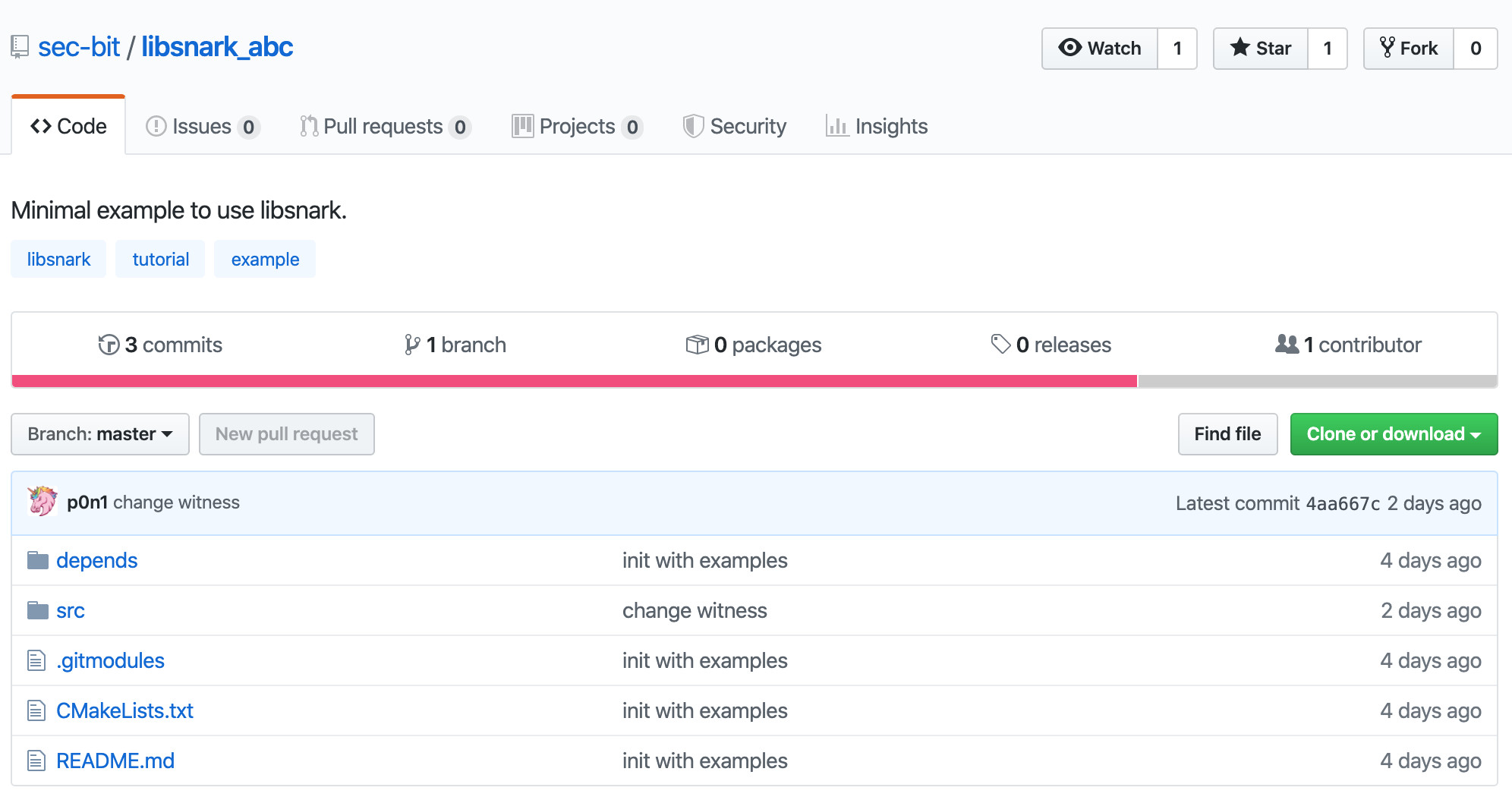通过 git submodule 拉取 libsnark 代码。

``````cd libsnark_abc
git submodule update --init --recursive``````

``sudo apt-get install build-essential cmake git libgmp3-dev libprocps4-dev python-markdown libboost-all-dev libssl-dev``

``mkdir build && cd build && cmake ..``

``mkdir build && cd build && CPPFLAGS=-I/usr/local/opt/openssl/include LDFLAGS=-L/usr/local/opt/openssl/lib PKG_CONFIG_PATH=/usr/local/opt/openssl/lib/pkgconfig cmake -DWITH_PROCPS=OFF -DWITH_SUPERCOP=OFF ..``

``make``

``````main
range
test``````

``./src/main``

## 4. 理解示例代码

``````r1cs_gg_ppzksnark_keypair&lt;ppT> keypair = r1cs_gg_ppzksnark_generator&lt;ppT>(example.constraint_system);
r1cs_gg_ppzksnark_processed_verification_key&lt;ppT> pvk = r1cs_gg_ppzksnark_verifier_process_vk&lt;ppT>(keypair.vk);
r1cs_gg_ppzksnark_proof&lt;ppT> proof = r1cs_gg_ppzksnark_prover&lt;ppT>(keypair.pk, example.primary_input, example.auxiliary_input);
const bool ans = r1cs_gg_ppzksnark_verifier_strong_IC&lt;ppT>(keypair.vk, example.primary_input, proof);
const bool ans2 = r1cs_gg_ppzksnark_online_verifier_strong_IC&lt;ppT>(pvk, example.primary_input, proof);``````

``````#include &lt;libsnark/common/default_types/r1cs_gg_ppzksnark_pp.hpp>
#include &lt;libsnark/zk_proof_systems/ppzksnark/r1cs_gg_ppzksnark/r1cs_gg_ppzksnark.hpp>
#include &lt;libsnark/gadgetlib1/pb_variable.hpp>``````

``````typedef libff::Fr&lt;default_r1cs_gg_ppzksnark_pp> FieldT;
default_r1cs_gg_ppzksnark_pp::init_public_params();``````

``````x * x = sym_1
sym_1 * x = y
y + x = sym_2
sym_2 + 5 = out``````

``````// Create protoboard
protoboard&lt;FieldT> pb;
// Define variables
pb_variable&lt;FieldT> x;
pb_variable&lt;FieldT> sym_1;
pb_variable&lt;FieldT> y;
pb_variable&lt;FieldT> sym_2;
pb_variable&lt;FieldT> out;``````

``````out.allocate(pb, "out");
x.allocate(pb, "x");
sym_1.allocate(pb, "sym_1");
y.allocate(pb, "y");
sym_2.allocate(pb, "sym_2");
pb.set_input_sizes(1);``````

``````// x*x = sym_1
pb.add_r1cs_constraint(r1cs_constraint&lt;FieldT>(x, x, sym_1));
// sym_1 * x = y
pb.add_r1cs_constraint(r1cs_constraint&lt;FieldT>(sym_1, x, y));
// y + x = sym_2
pb.add_r1cs_constraint(r1cs_constraint&lt;FieldT>(y + x, 1, sym_2));
// sym_2 + 5 = ~out
pb.add_r1cs_constraint(r1cs_constraint&lt;FieldT>(sym_2 + 5, 1, out));``````

``````const r1cs_constraint_system&lt;FieldT> constraint_system = pb.get_constraint_system();
const r1cs_gg_ppzksnark_keypair&lt;default_r1cs_gg_ppzksnark_pp> keypair = r1cs_gg_ppzksnark_generator&lt;default_r1cs_gg_ppzksnark_pp>(constraint_system);``````

``````pb.val(out) = 35;
pb.val(x) = 3;
pb.val(sym_1) = 9;
pb.val(y) = 27;
pb.val(sym_2) = 30;``````

``const r1cs_gg_ppzksnark_proof&lt;default_r1cs_gg_ppzksnark_pp> proof = r1cs_gg_ppzksnark_prover&lt;default_r1cs_gg_ppzksnark_pp>(keypair.pk, pb.primary_input(), pb.auxiliary_input());``

``bool verified = r1cs_gg_ppzksnark_verifier_strong_IC&lt;default_r1cs_gg_ppzksnark_pp>(keypair.vk, pb.primary_input(), proof);``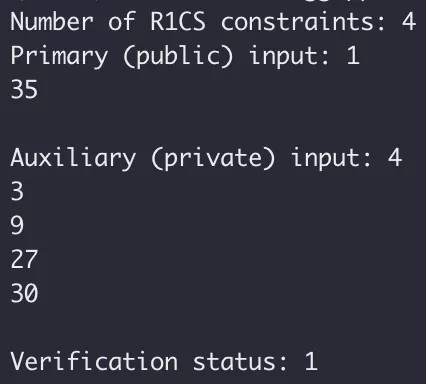## 5 再次上手实践

zk-SNARKs 电路开发的主要工作量和难点在于如何用代码“精确”地描述命题中的所有约束。一旦描述不“精确”，则要么是漏掉约束、要么是写错约束，最终电路想要证明的内容则会与原命题相差甚远。上一节的例子只涉及简单的乘法和加法，与 r1cs_constraint 最基本的形式一致，因此约束的表达相对容易。除此之外几乎所有的约束都不是很直观，作为初学者很难正确地描述约束细节。

DangDangDang，gadgetlib1/gadgets/basic_gadgets.hpp 中的 comparison_gadget 正是我们所需。

``````comparison_gadget(protoboard&lt;FieldT>& pb,
const size_t n,
const pb_linear_combination&lt;FieldT> &A,
const pb_linear_combination&lt;FieldT> &B,
const pb_variable&lt;FieldT> &less,
const pb_variable&lt;FieldT> &less_or_eq,
const std::string &annotation_prefix="")``````

``````protoboard&lt;FieldT> pb;
pb_variable&lt;FieldT> x, max;
pb_variable&lt;FieldT> less, less_or_eq;
x.allocate(pb, "x");
max.allocate(pb, "max");
pb.val(max)= 60;``````

``````comparison_gadget&lt;FieldT> cmp(pb, 10, x, max, less, less_or_eq, "cmp");
cmp.generate_r1cs_constraints();
pb.add_r1cs_constraint(r1cs_constraint&lt;FieldT>(less, 1, FieldT::one()));``````

``````// Add witness values
pb.val(x) = 18; // secret
cmp.generate_r1cs_witness();``````

## 7 附录

### main.cpp

``````#include &lt;libff/common/default_types/ec_pp.hpp>
#include &lt;libsnark/common/default_types/r1cs_gg_ppzksnark_pp.hpp>
#include &lt;libsnark/relations/constraint_satisfaction_problems/r1cs/examples/r1cs_examples.hpp>
#include &lt;libsnark/zk_proof_systems/ppzksnark/r1cs_gg_ppzksnark/r1cs_gg_ppzksnark.hpp>
using namespace libsnark;
/**
* The code below provides an example of all stages of running a R1CS GG-ppzkSNARK.
*
* Of course, in a real-life scenario, we would have three distinct entities,
* mangled into one in the demonstration below. The three entities are as follows.
* (1) The "generator", which runs the ppzkSNARK generator on input a given
*     constraint system CS to create a proving and a verification key for CS.
* (2) The "prover", which runs the ppzkSNARK prover on input the proving key,
*     a primary input for CS, and an auxiliary input for CS.
* (3) The "verifier", which runs the ppzkSNARK verifier on input the verification key,
*     a primary input for CS, and a proof.
*/
template&lt;typename ppT>
bool run_r1cs_gg_ppzksnark(const r1cs_example&lt;libff::Fr&lt;ppT> > &example)
{
libff::print_header("R1CS GG-ppzkSNARK Generator");
r1cs_gg_ppzksnark_keypair&lt;ppT> keypair = r1cs_gg_ppzksnark_generator&lt;ppT>(example.constraint_system);
printf("\n"); libff::print_indent(); libff::print_mem("after generator");
libff::print_header("Preprocess verification key");
r1cs_gg_ppzksnark_processed_verification_key&lt;ppT> pvk = r1cs_gg_ppzksnark_verifier_process_vk&lt;ppT>(keypair.vk);
libff::print_header("R1CS GG-ppzkSNARK Prover");
r1cs_gg_ppzksnark_proof&lt;ppT> proof = r1cs_gg_ppzksnark_prover&lt;ppT>(keypair.pk, example.primary_input, example.auxiliary_input);
printf("\n"); libff::print_indent(); libff::print_mem("after prover");
libff::print_header("R1CS GG-ppzkSNARK Verifier");
const bool ans = r1cs_gg_ppzksnark_verifier_strong_IC&lt;ppT>(keypair.vk, example.primary_input, proof);
printf("\n"); libff::print_indent(); libff::print_mem("after verifier");
printf("* The verification result is: %s\n", (ans ? "PASS" : "FAIL"));
libff::print_header("R1CS GG-ppzkSNARK Online Verifier");
const bool ans2 = r1cs_gg_ppzksnark_online_verifier_strong_IC&lt;ppT>(pvk, example.primary_input, proof);
assert(ans == ans2);
return ans;
}
template&lt;typename ppT>
void test_r1cs_gg_ppzksnark(size_t num_constraints, size_t input_size)
{
r1cs_example&lt;libff::Fr&lt;ppT> > example = generate_r1cs_example_with_binary_input&lt;libff::Fr&lt;ppT> >(num_constraints, input_size);
const bool bit = run_r1cs_gg_ppzksnark&lt;ppT>(example);
assert(bit);
}
int main () {
default_r1cs_gg_ppzksnark_pp::init_public_params();
test_r1cs_gg_ppzksnark&lt;default_r1cs_gg_ppzksnark_pp>(1000, 100);
return 0;
}``````

### test.cpp

``````#include &lt;libsnark/common/default_types/r1cs_gg_ppzksnark_pp.hpp>
#include &lt;libsnark/zk_proof_systems/ppzksnark/r1cs_gg_ppzksnark/r1cs_gg_ppzksnark.hpp>
#include &lt;libsnark/gadgetlib1/pb_variable.hpp>
using namespace libsnark;
using namespace std;
int main () {
typedef libff::Fr&lt;default_r1cs_gg_ppzksnark_pp> FieldT;
// Initialize the curve parameters
default_r1cs_gg_ppzksnark_pp::init_public_params();

// Create protoboard
protoboard&lt;FieldT> pb;
// Define variables
pb_variable&lt;FieldT> x;
pb_variable&lt;FieldT> sym_1;
pb_variable&lt;FieldT> y;
pb_variable&lt;FieldT> sym_2;
pb_variable&lt;FieldT> out;
// Allocate variables to protoboard
// The strings (like "x") are only for debugging purposes
out.allocate(pb, "out");
x.allocate(pb, "x");
sym_1.allocate(pb, "sym_1");
y.allocate(pb, "y");
sym_2.allocate(pb, "sym_2");
// This sets up the protoboard variables
// so that the first one (out) represents the public
// input and the rest is private input
pb.set_input_sizes(1);
// Add R1CS constraints to protoboard
// x*x = sym_1
pb.add_r1cs_constraint(r1cs_constraint&lt;FieldT>(x, x, sym_1));
// sym_1 * x = y
pb.add_r1cs_constraint(r1cs_constraint&lt;FieldT>(sym_1, x, y));
// y + x = sym_2
pb.add_r1cs_constraint(r1cs_constraint&lt;FieldT>(y + x, 1, sym_2));
// sym_2 + 5 = ~out
pb.add_r1cs_constraint(r1cs_constraint&lt;FieldT>(sym_2 + 5, 1, out));

const r1cs_constraint_system&lt;FieldT> constraint_system = pb.get_constraint_system();
// generate keypair
const r1cs_gg_ppzksnark_keypair&lt;default_r1cs_gg_ppzksnark_pp> keypair = r1cs_gg_ppzksnark_generator&lt;default_r1cs_gg_ppzksnark_pp>(constraint_system);
// Add public input and witness values
pb.val(out) = 35;
pb.val(x) = 3;
pb.val(sym_1) = 9;
pb.val(y) = 27;
pb.val(sym_2) = 30;
// generate proof
const r1cs_gg_ppzksnark_proof&lt;default_r1cs_gg_ppzksnark_pp> proof = r1cs_gg_ppzksnark_prover&lt;default_r1cs_gg_ppzksnark_pp>(keypair.pk, pb.primary_input(), pb.auxiliary_input());
// verify
bool verified = r1cs_gg_ppzksnark_verifier_strong_IC&lt;default_r1cs_gg_ppzksnark_pp>(keypair.vk, pb.primary_input(), proof);
cout &lt;&lt; "Number of R1CS constraints: " &lt;&lt; constraint_system.num_constraints() &lt;&lt; endl;
cout &lt;&lt; "Primary (public) input: " &lt;&lt; pb.primary_input() &lt;&lt; endl;
cout &lt;&lt; "Auxiliary (private) input: " &lt;&lt; pb.auxiliary_input() &lt;&lt; endl;
cout &lt;&lt; "Verification status: " &lt;&lt; verified &lt;&lt; endl;
}``````

### range.cpp

``````#include &lt;libsnark/common/default_types/r1cs_gg_ppzksnark_pp.hpp>
#include &lt;libsnark/zk_proof_systems/ppzksnark/r1cs_gg_ppzksnark/r1cs_gg_ppzksnark.hpp>
#include &lt;libsnark/gadgetlib1/pb_variable.hpp>
#include &lt;libsnark/gadgetlib1/gadgets/basic_gadgets.hpp>
using namespace libsnark;
using namespace std;
int main () {
typedef libff::Fr&lt;default_r1cs_gg_ppzksnark_pp> FieldT;
// Initialize the curve parameters
default_r1cs_gg_ppzksnark_pp::init_public_params();

// Create protoboard
protoboard&lt;FieldT> pb;
pb_variable&lt;FieldT> x, max;
pb_variable&lt;FieldT> less, less_or_eq;
x.allocate(pb, "x");
max.allocate(pb, "max");

pb.val(max)= 60;
comparison_gadget&lt;FieldT> cmp(pb, 10, x, max, less, less_or_eq, "cmp");
cmp.generate_r1cs_constraints();
pb.add_r1cs_constraint(r1cs_constraint&lt;FieldT>(less, 1, FieldT::one()));
const r1cs_constraint_system&lt;FieldT> constraint_system = pb.get_constraint_system();
// generate keypair
const r1cs_gg_ppzksnark_keypair&lt;default_r1cs_gg_ppzksnark_pp> keypair = r1cs_gg_ppzksnark_generator&lt;default_r1cs_gg_ppzksnark_pp>(constraint_system);
// Add witness values
pb.val(x) = 18; // secret
cmp.generate_r1cs_witness();
// generate proof
const r1cs_gg_ppzksnark_proof&lt;default_r1cs_gg_ppzksnark_pp> proof = r1cs_gg_ppzksnark_prover&lt;default_r1cs_gg_ppzksnark_pp>(keypair.pk, pb.primary_input(), pb.auxiliary_input());
// verify
bool verified = r1cs_gg_ppzksnark_verifier_strong_IC&lt;default_r1cs_gg_ppzksnark_pp>(keypair.vk, pb.primary_input(), proof);
cout &lt;&lt; "Number of R1CS constraints: " &lt;&lt; constraint_system.num_constraints() &lt;&lt; endl;
cout &lt;&lt; "Primary (public) input: " &lt;&lt; pb.primary_input() &lt;&lt; endl;
cout &lt;&lt; "Auxiliary (private) input: " &lt;&lt; pb.auxiliary_input() &lt;&lt; endl;
cout &lt;&lt; "Verification status: " &lt;&lt; verified &lt;&lt; endl;
}``````

• 发表于 2020-01-03 15:40
• 阅读 ( 1036 )
• 学分 ( 35 )
• 分类：零知识证明